# 第2章 静力学--课后作业【含答案】 水力学

（1）单选题

A  压应力

B  压应力和拉应力

C  压应力和切应力

D  压应力、切应力和拉应力

（2）多选题

A  流体静压强垂直于作用面，属于表面力

B  流体静压强存在于流体内部，同一点上各个方向都存在，属于质量力

C  流体静压强是标量

D  流体静压强是矢量

（3）多选题

A  铅垂方向上的压强变化与深度变化是线性关系

B  水平方向上的压强不变化

C  铅垂方向上压强随深度的变化快慢仅与重力加速度有关

D  铅垂方向上压强随深度的变化快慢与流体的种类和重力加速度有关

（4）单选题

A  切应力不变

B  压强不变

C  压强增加

D  压强减少

（5）单选题

A  对

B  错

（6）单选题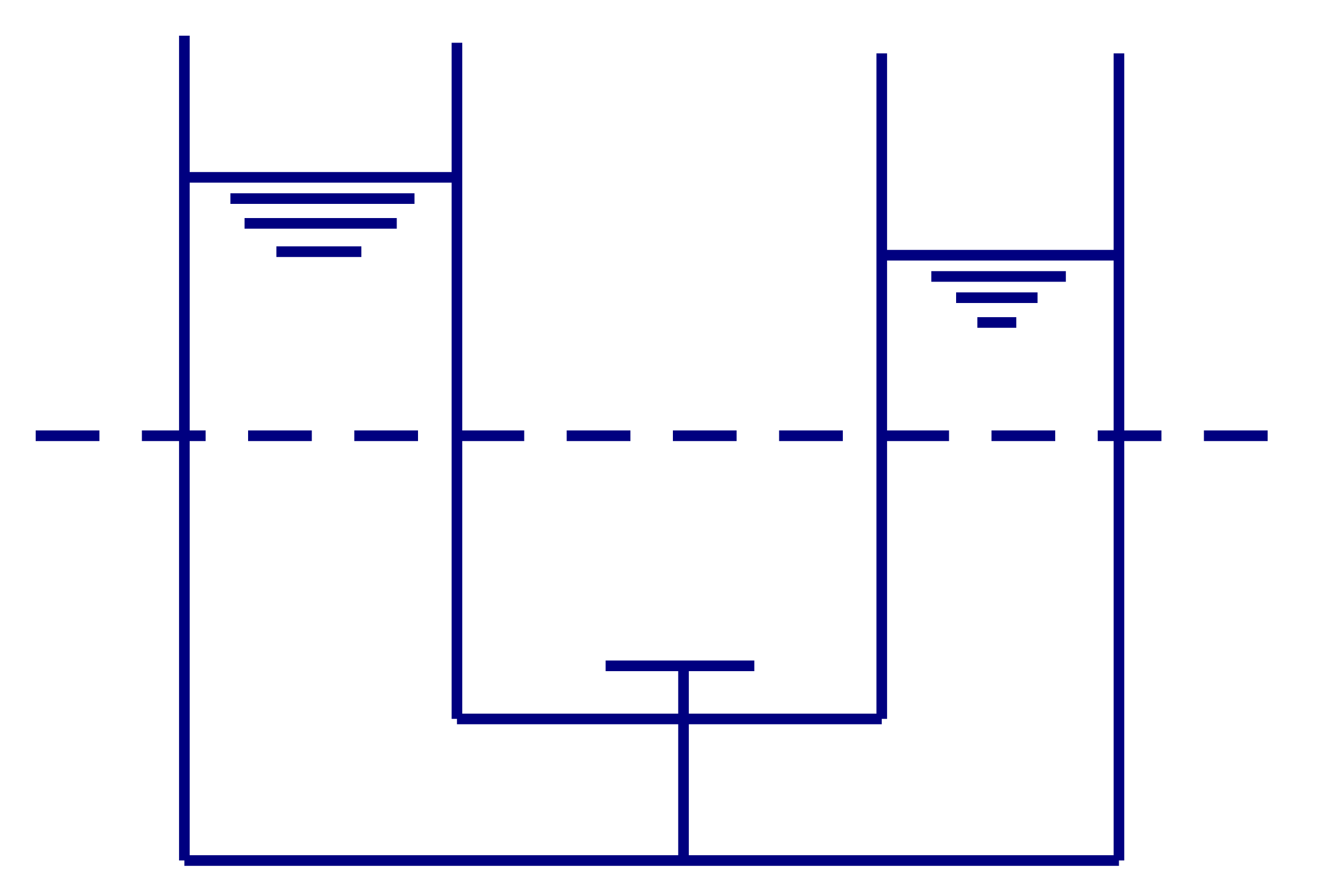A  对

B  错

（7）单选题

A  52000

B  49325

C  48000

D  -48000

（8）单选题

A.$p_1=p_2=p_3$

B.$p_1 \gt p_2 \gt p_3$

C.$p_1 \lt p_2 \lt p_3$

D.$p_1 \lt p_2 = p_3$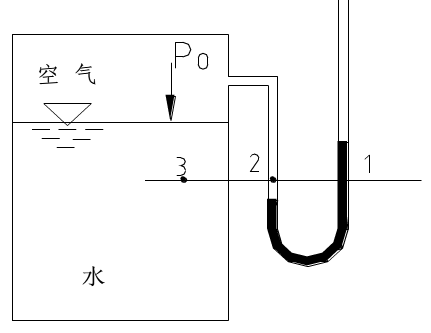A  A

B  B

C  C

D  D

（9）单选题

A  45°倾斜

B  60°倾斜

C  水平

D  铅直

（10）单选题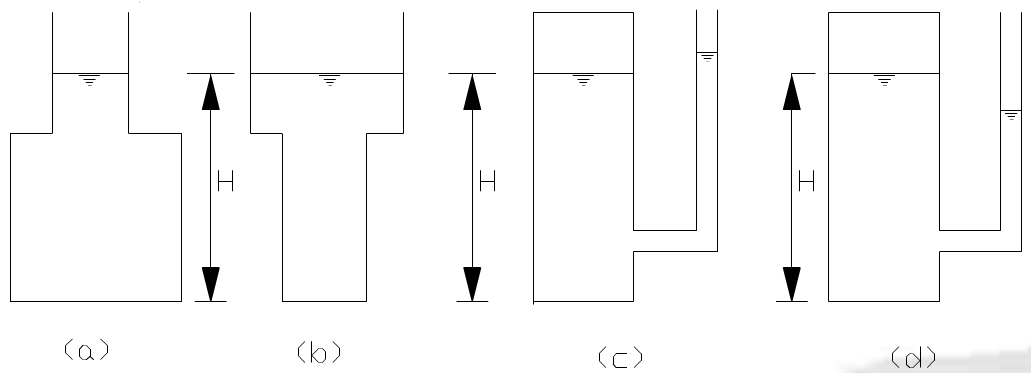A  （a）

B  （b）

C  （c）

D  （d）

（11）单选题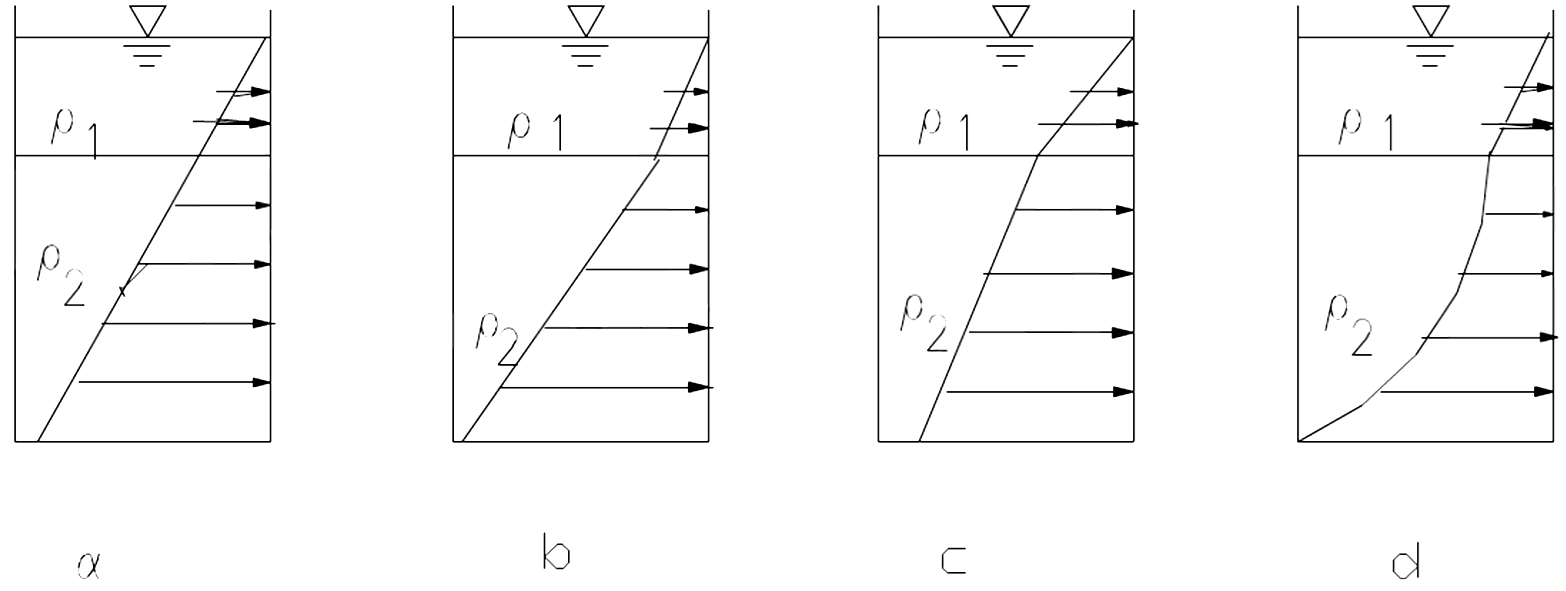A  A

B  B

C  C

D  D

（12）单选题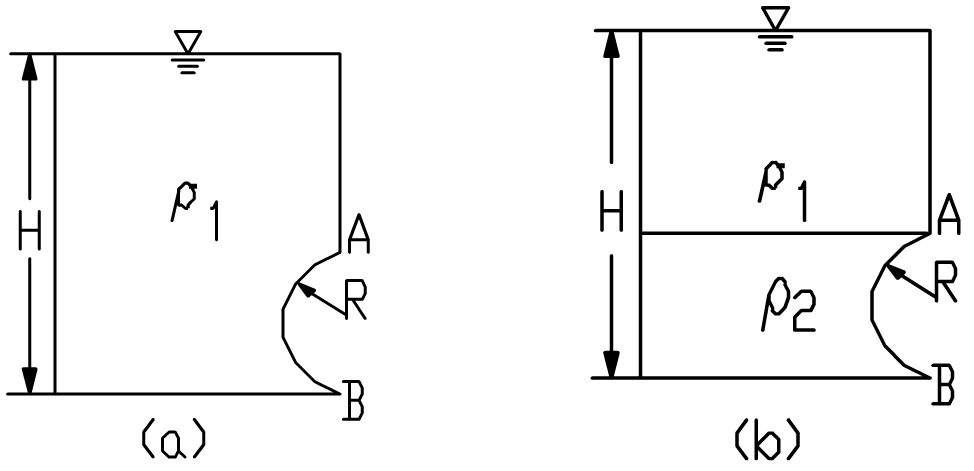A  压力体相同，且压力相等

B  压力体相同，但压力不等

C  压力体不同，且压力不等

D  压力体不同，但压力相等

（13）单选题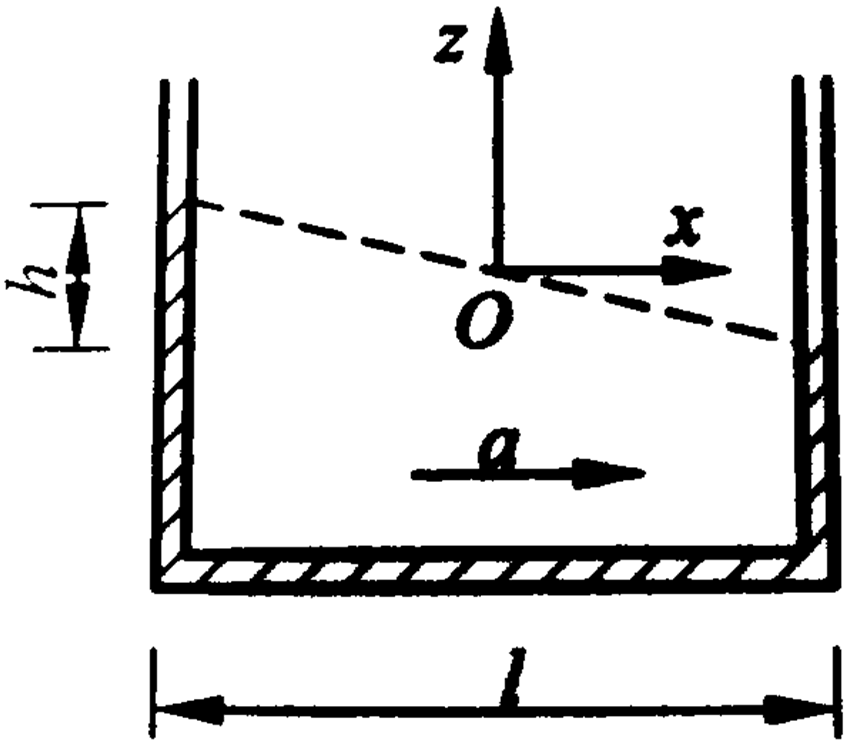A  流体的加速度为a=g

B  流体的加速度为a=gh/l

C  流体的加速度为a=gl/h

D  仅有h和l不能确定流体的加速度a